GeeksforGeeks App
Open AppBrowser
Continue

# Microsoft Azure – Track CPU Utilization of a Azure Virtual Machines using KQL Log Query

In this article, we will look into the process of finding the Minimum, Average, and Maximum CPU Utilization of all Azure Virtual Machines using KQL Log Query.

Here the Perf KQL Counter Operator is used to find Azure Virtual Machines performance from collected data logs.

## Implementation:

Follow the below steps to Run the Log Queries.

Step 2: Search/Go to Log Analytics Workspace and select your Log Analytics Workspace. Create one if you don’t have any and enable agent configurations.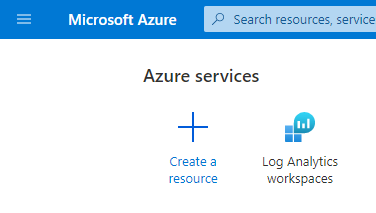• Then, from the left menu navigate to General >> Logs.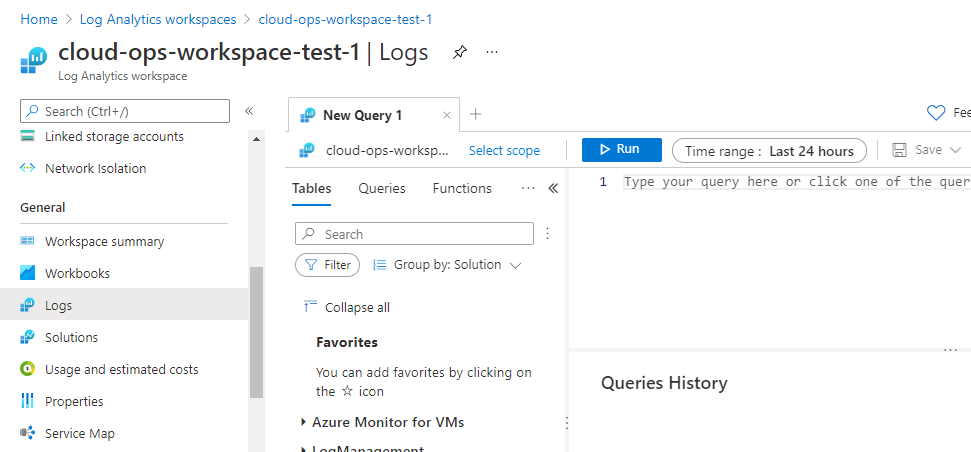Now, Paste the below Queries to get the log data.

Before Running the Query understand the Query Syntax.

Explanation for all the below examples :

Here, Perf represents performance, this is an operator which is used to fetch the performance logs of Azure Compute resources like Azure VMs, SQL Servers, Disk Storage etc.

We are using the ObjectName == “Processor” and CounterName == “% Processor Time” both these conditions filter the process components of Windows and Linux VMs using a where keyword.

TimeGenerated > ago(7d) or TimeGenerated > ago(5h) helps in filter the logs collected within that timespan.

With summarize keyword we are rendering the required data in the form of a table chart.

### KQL Queries for Analysing CPU Performance of Azure VMs.

Example: To find Minimum CPU Utilization of Azure Virtual Machines for the last 7 days.

```Perf
| where ObjectName == "Processor" and CounterName == "% Processor Time" and InstanceName == "_Total"
| where TimeGenerated > ago(7d)
| summarize MIN_CPU = min(CounterValue) by Computer, _ResourceId```

Output: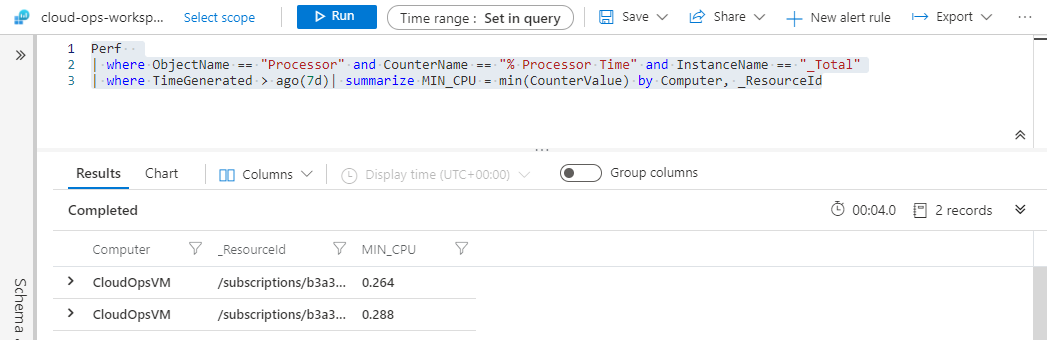Example: To find Minimum CPU Utilization of Azure Virtual Machines for the last 5 hours

```Perf
| where ObjectName == "Processor" and CounterName == "% Processor Time" and InstanceName == "_Total"
| where TimeGenerated > ago(5h)
| summarize MIN_CPU = min(CounterValue) by  Computer, _ResourceId```

Output: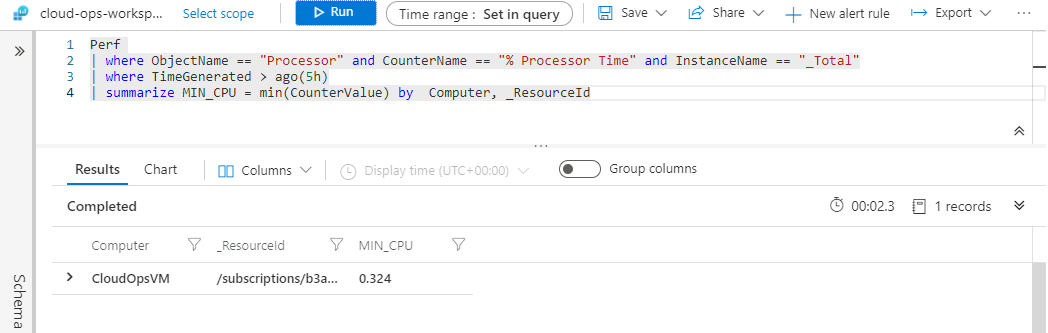Example: To find Average CPU Utilization of Azure Virtual Machines for the last 7 days

```Perf
| where ObjectName == "Processor" and CounterName == "% Processor Time" and InstanceName == "_Total"
| where TimeGenerated > ago(7d)
| summarize AVG_CPU = avg(CounterValue) by  Computer, _ResourceId```

Output: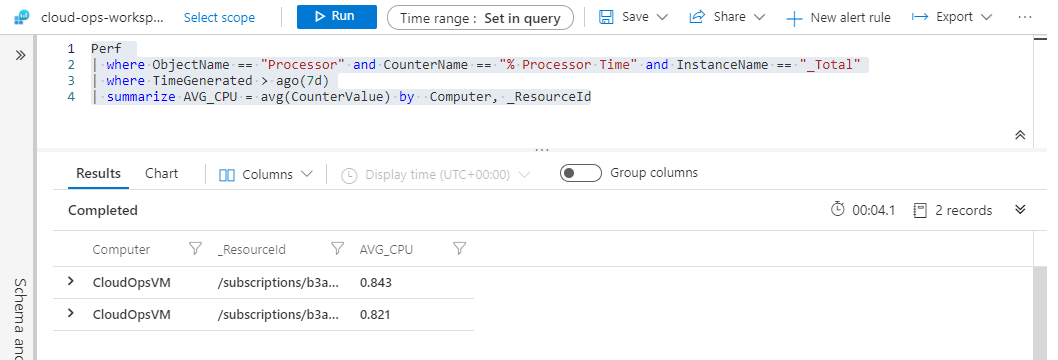Example: To find Average  CPU Utilization of Azure Virtual Machines for the last 5 hours.

```Perf
| where ObjectName == "Processor" and CounterName == "% Processor Time" and InstanceName == "_Total"
| where TimeGenerated > ago(5h)
| summarize AVG_CPU = avg(CounterValue) by  Computer, _ResourceId```

Output: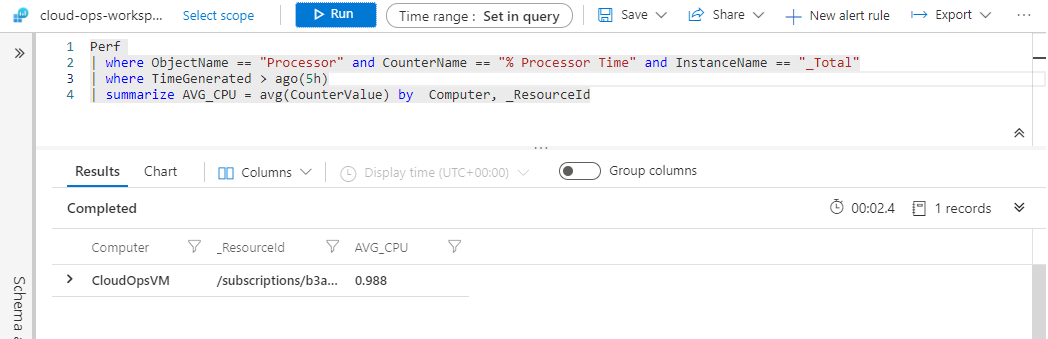Example: To find Maximum CPU Utilization of Azure Virtual Machines for the last 7 days.

```Perf
| where ObjectName == "Processor" and CounterName == "% Processor Time" and InstanceName == "_Total"
| where TimeGenerated > ago(7d)
| summarize MAX_CPU = max(CounterValue) by  Computer, _ResourceId```

Output: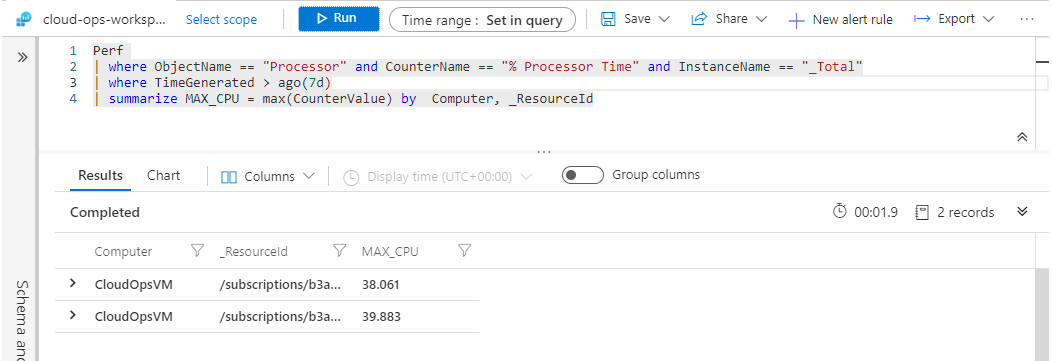Example: To find Maximum CPU Utilization of Azure Virtual Machines for the last 5 hours.

```Perf
| where ObjectName == "Processor" and CounterName == "% Processor Time" and InstanceName == "_Total"
| where TimeGenerated > ago(2h)
| summarize MAX_CPU = max(CounterValue) by Computer, _ResourceId```

Output: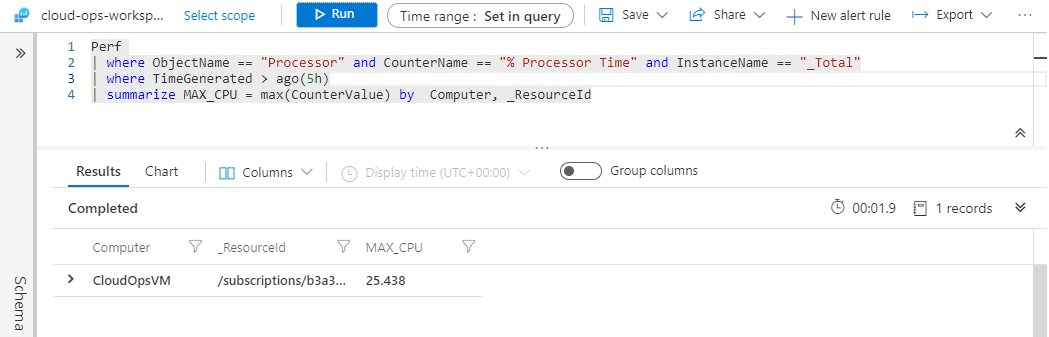Example: To analyze VM CPU Utilization of Minimum, Average and Maximum for last 7 days in a single table chart.

```Perf
| where ObjectName == "Processor" and CounterName == "% Processor Time" and InstanceName == "_Total"
| where TimeGenerated > ago(7d)
| summarize MIN_CPU = min(CounterValue), AVG_CPU = avg(CounterValue), MAX_CPU = max(CounterValue) by  Computer, _ResourceId```

Output: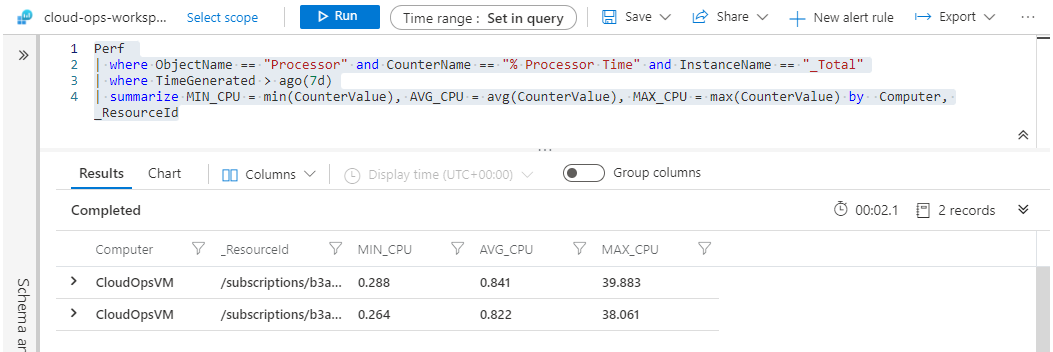Example: To analyze VM CPU Utilization of Minimum, Average and Maximum for last 5 hours in a single table chart.

```Perf
| where ObjectName == "Processor" and CounterName == "% Processor Time" and InstanceName == "_Total"
| where TimeGenerated > ago(5h)
| summarize MIN_CPU = min(CounterValue), AVG_CPU = avg(CounterValue), MAX_CPU = max(CounterValue) by  Computer, _ResourceId```

Output: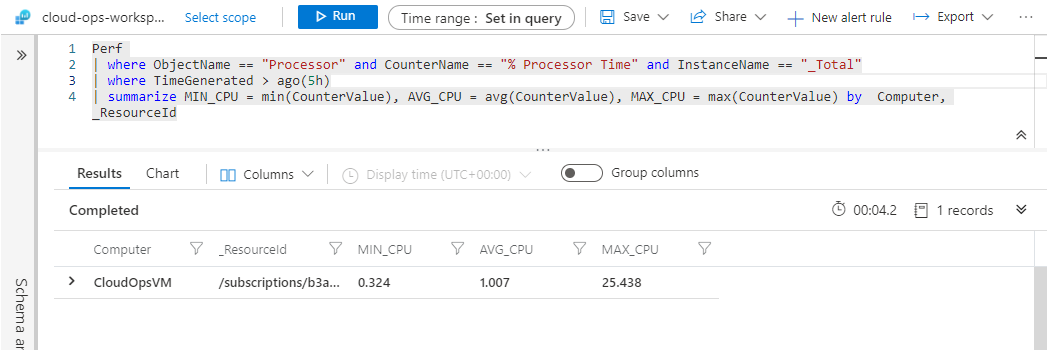Note: This process works for both the OS Types. i.e., Windows and Linux.

That’s it we are done.

My Personal Notes arrow_drop_up
Related Tutorials